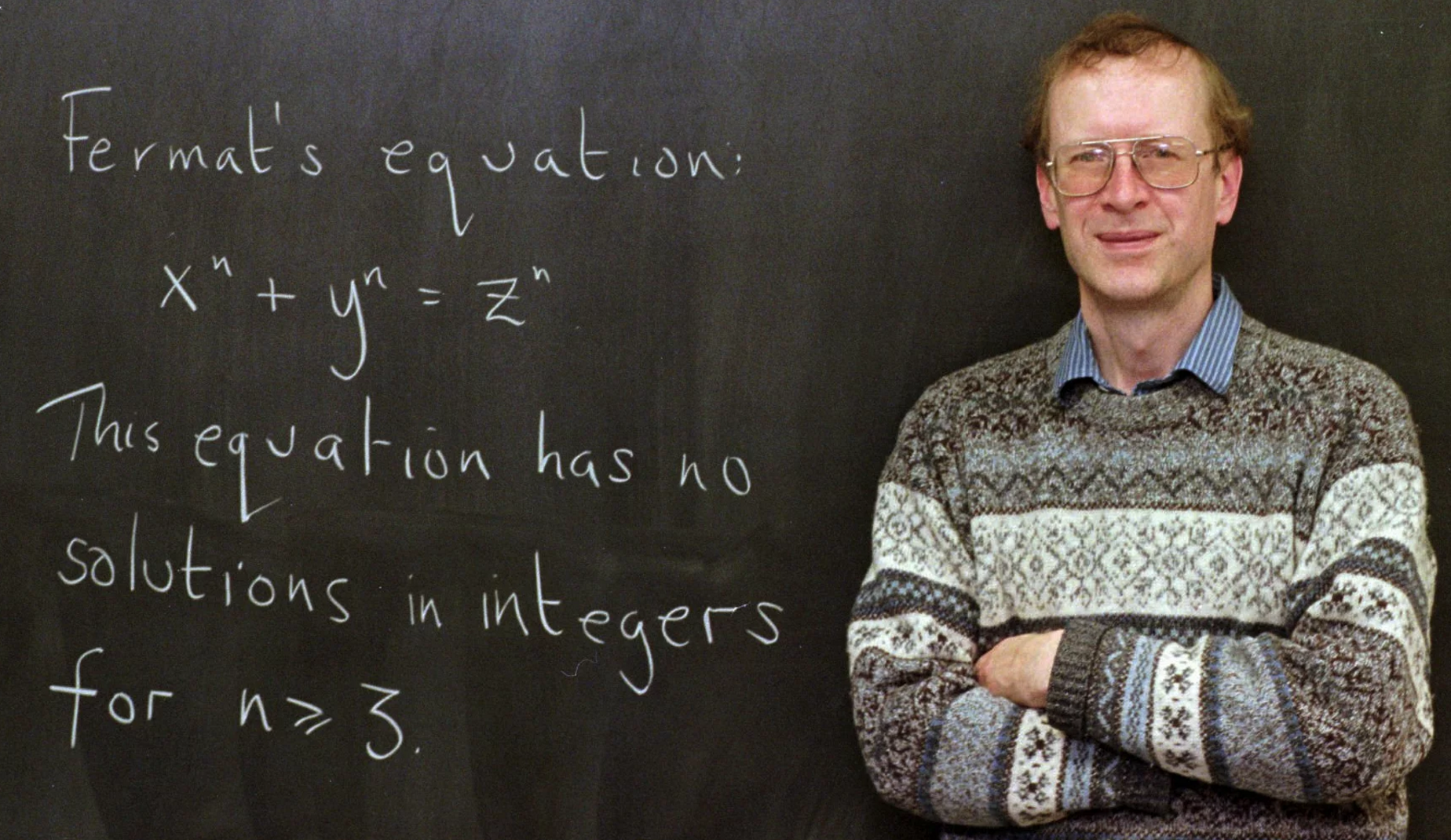# Fermat's Last TheoremAndrew Wiles, pictured above, was recently awarded the 2016 Abel Prize for his 1994 proof of Fermat's last theorem.

Suppose $n=2016$ and $|x|,|y|,|z|$ are all less than 10. How many solutions in integers are there to the equation on the chalkboard?

Note: A solution here refers to an ordered triple $(x,y,z).$

×